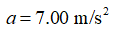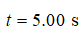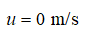# 18. A car starts from rest and travels in a straight line with a constant acceleration of 7.00 m/s^2. After a time of 5.00 s, which of these expressions yields how far the car traveled from its starting point in meters? a.7.00 times 5.00 b.½ times 7.00 times 5.00^2

Question
1 views
18. A car starts from rest and travels in a straight line with a constant acceleration of 7.00 m/s^2. After a time of 5.00 s, which of these expressions yields how far the car traveled from its starting point in meters?
 a. 7.00 times 5.00 b. ½ times 7.00 times 5.00^2
check_circle

Step 1

The acceleration of the car,Step 2

Time taken,Step 3

The car starts from the rest.

The initial speed of the car,...

### Want to see the full answer?

See Solution

#### Want to see this answer and more?

Solutions are written by subject experts who are available 24/7. Questions are typically answered within 1 hour.*

See Solution
*Response times may vary by subject and question.
Tagged in